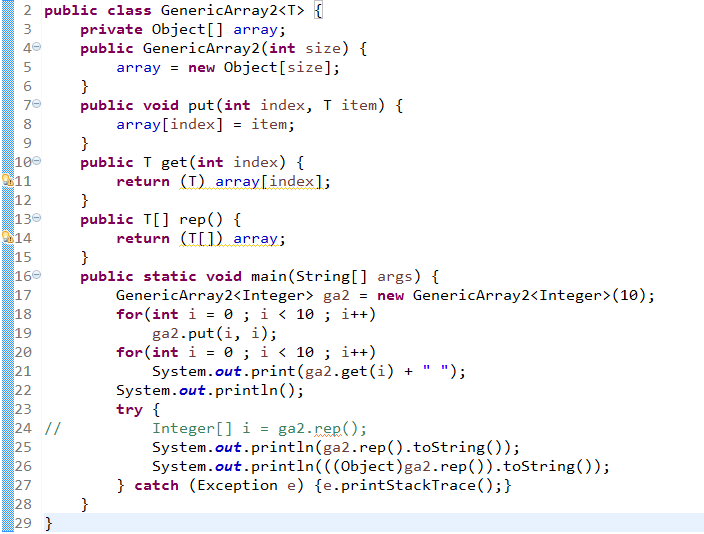JAVA泛型的问题：急求大神。一个关于"泛型"和Object[]数组的坑public class GenericArray {

``````private Object[] array;

public GenericArray(int size) {
array = new Object[size];
}

public void put(int index, T item) {
array[index] = item;
}

public T get(int index) {
return (T) array[index];
}

public T[] rep() {
return (T[]) array;
}

public static void main(String[] args) {
GenericArray<Integer> ga = new GenericArray<>(10);
for(int i = 0; i < 10; i++) {
ga.put(i, i);
}
for(int i = 0; i < 10; i++) {
System.out.println(ga.get(i) + " ");
}
System.out.println();
try {
System.out.println(ga.rep().toString());
System.out.println(((Object)ga.rep()).toString());
} catch(Exception e) {
e.printStackTrace();
}
}
``````

}

8个回答2 年多之前 回复2 年多之前 回复2 年多之前 回复
``````    @Test
public void test(){
Integer[] ii = new Integer[]{};
Object[] oo = new Object[]{};
ii = (Integer[])oo;
}

``````luaifei2008 回复外星喵: 在Java中数组是具有协变性的，Integer[]是Object[]的子类，Java认为没毛病~
2 年多之前 回复2 年多之前 回复2 年多之前 回复2 年多之前 回复2 年多之前 回复2 年多之前 回复2 年多之前 回复

0 1 2 3 4 5 6 7 8 9
GenericArray [array=[0, 1, 2, 3, 4, 5, 6, 7, 8, 9]]

`````` import java.util.Arrays;

public class GenericArray<T> {
private T[] array;

public GenericArray(int size) {
array = (T[]) new Object[size];
}

public void put(int index, T item) {
array[index] = item;
}

public T get(int index) {
return array[index];
}

@Override
public String toString() {
return "GenericArray [array=" + Arrays.toString(array) + "]";
}

public static void main(String[] args) {
GenericArray<Integer> ga = new GenericArray<>(10);
for(int i = 0; i < 10; i++) {
ga.put(i, i);
}
for(int i = 0; i < 10; i++) {
System.out.print(ga.get(i) + " ");
}
System.out.println();
try {
System.out.println(ga.toString());
} catch(Exception e) {
e.printStackTrace();
}
}
}
``````2 年多之前 回复

Object[] o是确定的类型，T[] t是泛型，编译的时候编译期不知道T[]是什么类型，而把泛型擦除，即把T[]变为Object[]（Object[]是父类数组没问题），所以编译器只是给出警告2 年多之前 回复luaifei2008 回复菩提本无树明镜亦非台: 由于泛型擦除的原因，rep()方法实际返回的是Object[]数组，并非Integer[]数组，从报错可以看到是Object[]转Integer[]失败。至于为什么会存在类型转换这一步，目前还没有考虑清楚。
2 年多之前 回复2 年多之前 回复luaifei2008 也就是说，泛型的类型转换T[] array，不管泛型为何种类型，最后都会变成Object[] array，Object[]是父类数组肯定是没问题的，所以编译器只会警告提醒。
2 年多之前 回复

3行定义，改成T[] array2 年多之前 回复Java通过反射实例化泛型类的问题

Java语言中，想通过反射实例化一个抽象类的子类，问题也可以简化点，可以建个类继承这个抽象类，然后实例化子类。 [code="java"] public abstract class MyClass<T> { MyClass() { } } [/code] 在代码中可以这么写： [code="java"] new MyClass<String>() {} [/code] 但是如果通过反射，怎么把String这个type传进去，或者通过反射获得constructor，怎么把泛型信息传进去： 下面这个是个错误的写法，只是说明要实现的内容： [code="java"] new MyClass<Class.forName("String")>() { } [/code]

java泛型问题，方法参数问题

Java泛型. 代码如下： class Info<T>{ private T var; public void setVar(T var){ this.var=var; } public T getVar(){ return this.var; } public String toString(){ return this.var.toString(); } } 下面是第一个测试程序： public class GenericsDemo6{ public static void main(String []agrs){ Info info=new Info(); info.setVar("lixinghua"); String str=(String)info.getVar(); System.out.println(info.getVar()); } } 第二个测试程序； public class GenericsDemo8{ public static void main(String []agrs){ Info<String> info=new Info<String>(); fun(info); } public static void fun(Info<Object> info){ System.out.println(info.getVar()); } } 为什么第一个测试程序可以运行，而第二个不可以？第一个测试程序没有指定泛型，则默认是Object，这里String可以向上转型为Object,而第二个程序却不可以向上转型为Object？

java泛型问题，定义泛型方法

java泛型中，定义泛型方法格式:public <T> T fun(T t),这几个T分别是什么意思？ 我知道最后一个T是参数，那前面两个T表示什么？哪个表示返回类型？求高手指教

java 泛型擦除发生在哪个阶段，如何用反编译工具查看泛型擦除后的代码？

java泛型当中String 和Object之间是什么关系

Map<String, Object>里面的参数可以反过来写吗 写成Map <Object,String>

Java泛型问题，空指针问题

Java类的泛型参数能作为另外一个泛型类的参数传入吗？

JAVA_数组_泛型：我写了一个数组结合泛型的通用求和方法，求帮忙优化，写的太烂了

=====《求求大神帮我优化下啊，我写的太烂了》==== public static void main(String[] args) { Number[] num1 = {2, 3, 3, 4, 5}; //Integer Number[] num2 = {1.1, 2.0, 3.0, 4.0, 5.0}; //Double System.out.println(num1.getClass().getTypeName()); System.out.println(new ArraysTest().ArraysSum(num2)); } public static <T extends Number> T ArraysSum(T[] num) { System.out.println(num.getClass().getTypeName()); Double sum = 0.0; for (int i = 0; i < num.length; i++) { sum += num[i].doubleValue(); } if (num.getClass().getTypeName().equals("java.lang.Integer")){ Integer result = (int)((double)sum); return (T)result; } if (num.getClass().getTypeName().equals("java.lang.Double")){ Double result = sum.doubleValue(); return (T)result; } return (T)sum; } 如何结合instanceof 更加智能的判断？

java泛型＜？>什么泛型参数

java泛型＜？>什么泛型参数，是不是无视它，假如可以无视它，为什么还要写上它

public static void main(String[] args) { //告诉编译器我这个集合只能存储String类型的 List<String> col=new ArrayList<String>(); //告诉编译器我这个集合只能存储Integer类型的 List<Integer> col2=new ArrayList<Integer>(); //输出结果为：true //这就是常说的去除，编译后jvm虚拟机不会知道这个集合存储什么类型的值 System.out.println(col.getClass()==col2.getClass()); //看我如何将String存储到集合col2中。 /** * 在运行过程中，jvm根本不知道col2应该存放什么类型的数据，只是我们在编译器中限定了。 * 我们通过反射可以获取list的add方法给col2存储其他类型的数据。如这里就可以将“abc”存放到col2中 * 不明白这里为什么参数一定要是Object.class，不能改为String.class */ try { col2.getClass().getMethod("add", Object.class).invoke(col2, "abc"); System.out.println(col2.get(0)); } catch (IllegalArgumentException e) { // TODO Auto-generated catch block e.printStackTrace(); } catch (SecurityException e) { // TODO Auto-generated catch block e.printStackTrace(); } catch (IllegalAccessException e) { // TODO Auto-generated catch block e.printStackTrace(); } catch (InvocationTargetException e) { // TODO Auto-generated catch block e.printStackTrace(); } catch (NoSuchMethodException e) { // TODO Auto-generated catch block e.printStackTrace(); } } ``` ``` 为什么这里必须是Object.class了，list里的add是泛型啊。col2.getClass().getMethod("add", Object.class)

java泛型异常，如何解决

public static <AnyType extends Comparable<? super AnyType>> AnyType[] mergesort(AnyType[] a,AnyType[] b) { int i=0; int j=0; int index=0; AnyType[] tmp=(AnyType[]) new Comparable[a.length+b.length]; while(i<a.length&&j<b.length){ if(a[i].compareTo(b[j])<0) { tmp[index]=a[i]; i++; index++; } else{ tmp[index]=b[j]; j++; index++; } } return tmp; } public static void main(String[] args){ Integer a[]={1,13,24,26}; Integer b[]={2,15,27,28}; Integer[] tmp= (Integer[])mergesort(a, b); System.out.println(tmp); } } 结果：Exception in thread "main" java.lang.ClassCastException: [Ljava.lang.Comparable; cannot be cast to [Ljava.lang.Integer; at testcc.mergeSort.main(mergeSort.java:58) 求问如何解决

Java 泛型类型转换问题

java 泛型类的构造函数，急急！！！

dubbo rpc如何指定fastjson方式序列化参数对象

java泛型中的自限定类型有什么作用

java泛型中的自限定类型有什么作用？具体举个例子，一个应用场景

ArrayList源码分析(入门篇)

ArrayList源码分析 前言： 写这篇博客的主要原因是，在我上一次参加千牵科技Java实习生面试时，有被面试官问到ArrayList为什么查找的速度较快，插入和删除的速度较慢？当时我回答得不好，很大的一部分原因是因为我没有阅读过ArrayList源码，虽然最后收到Offer了，但我拒绝了，打算寒假学得再深入些再广泛些，下学期开学后再去投递其他更好的公司。为了更加深入理解ArrayList，也为

String s = new String(" a ") 到底产生几个对象？

loonggg读完需要3分钟速读仅需 1 分钟大家好，我是你们的校长。我之前讲过，这年头，只要肯动脑，肯行动，程序员凭借自己的技术，赚钱的方式还是有很多种的。仅仅靠在公司出卖自己的劳动时...

MySQL数据库面试题（2020最新版）

HashMap底层实现原理，红黑树，B+树，B树的结构原理 Spring的AOP和IOC是什么？它们常见的使用场景有哪些？Spring事务，事务的属性，传播行为，数据库隔离级别 Spring和SpringMVC，MyBatis以及SpringBoot的注解分别有哪些？SpringMVC的工作原理，SpringBoot框架的优点，MyBatis框架的优点 SpringCould组件有哪些，他们...

《经典算法案例》01-08：如何使用质数设计扫雷（Minesweeper）游戏